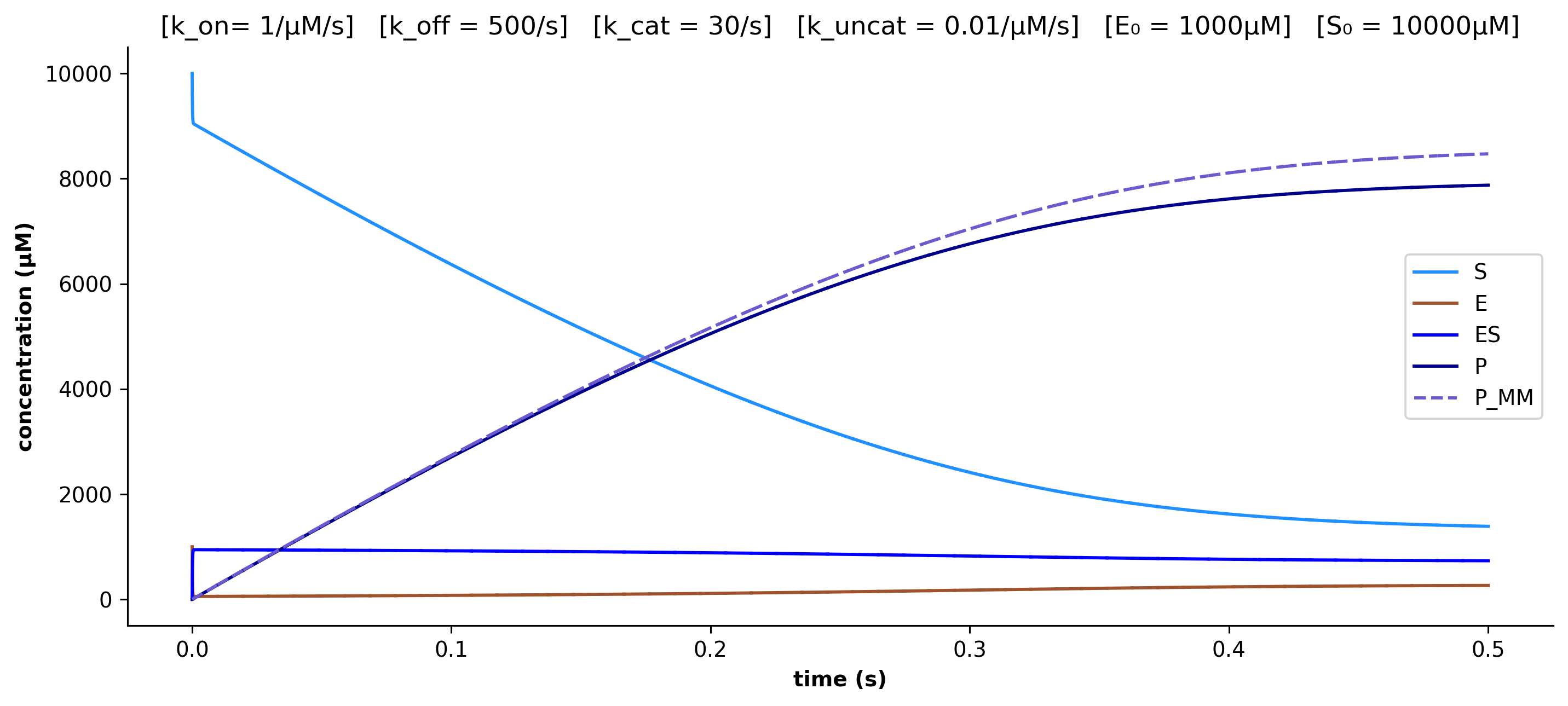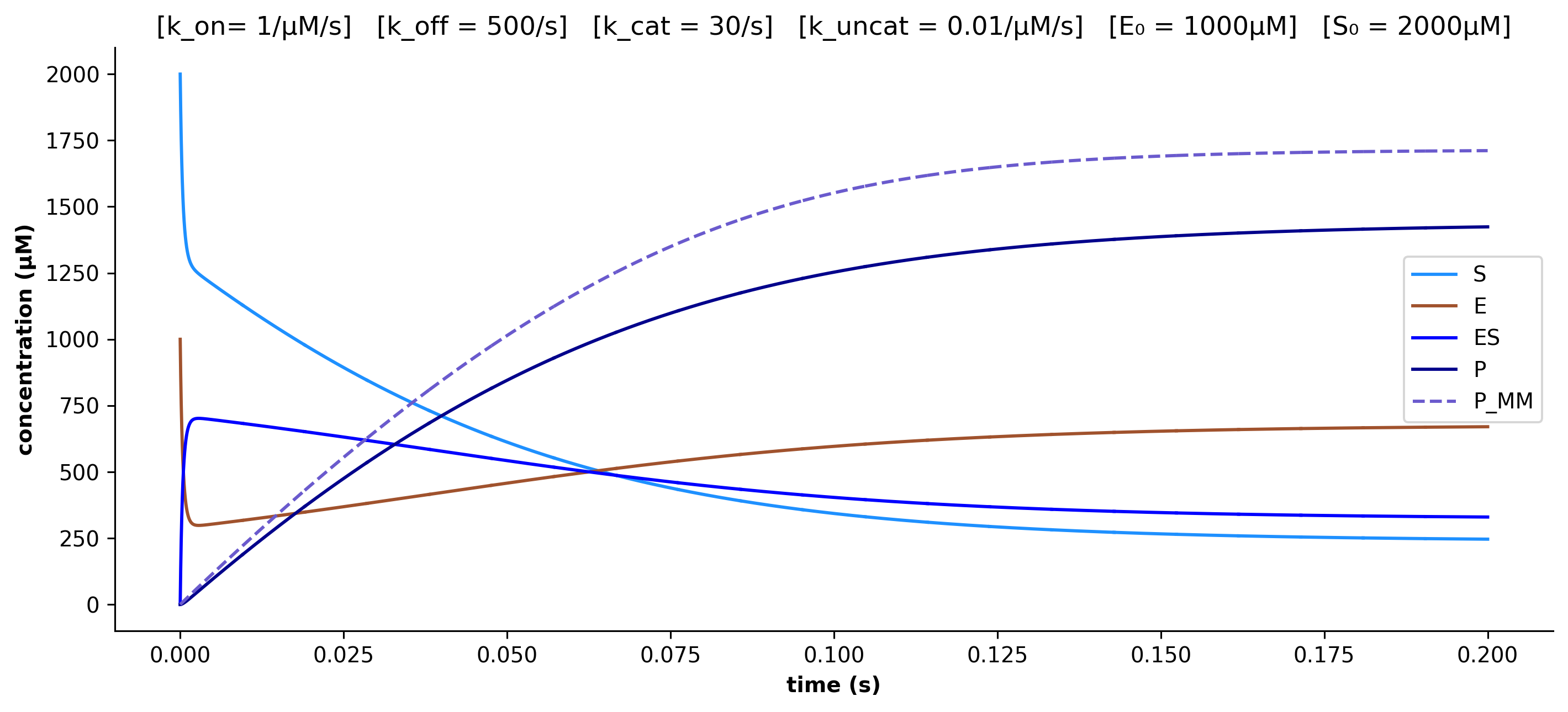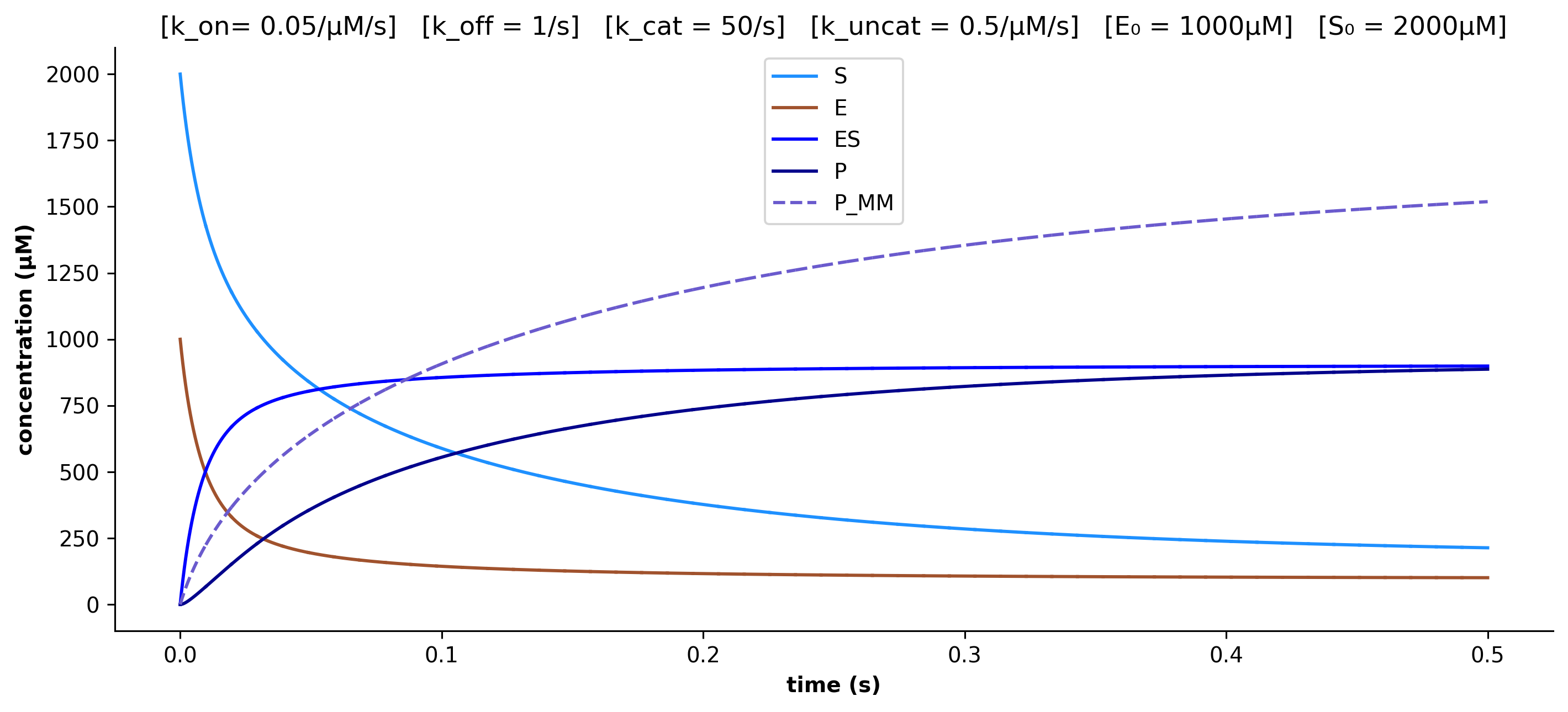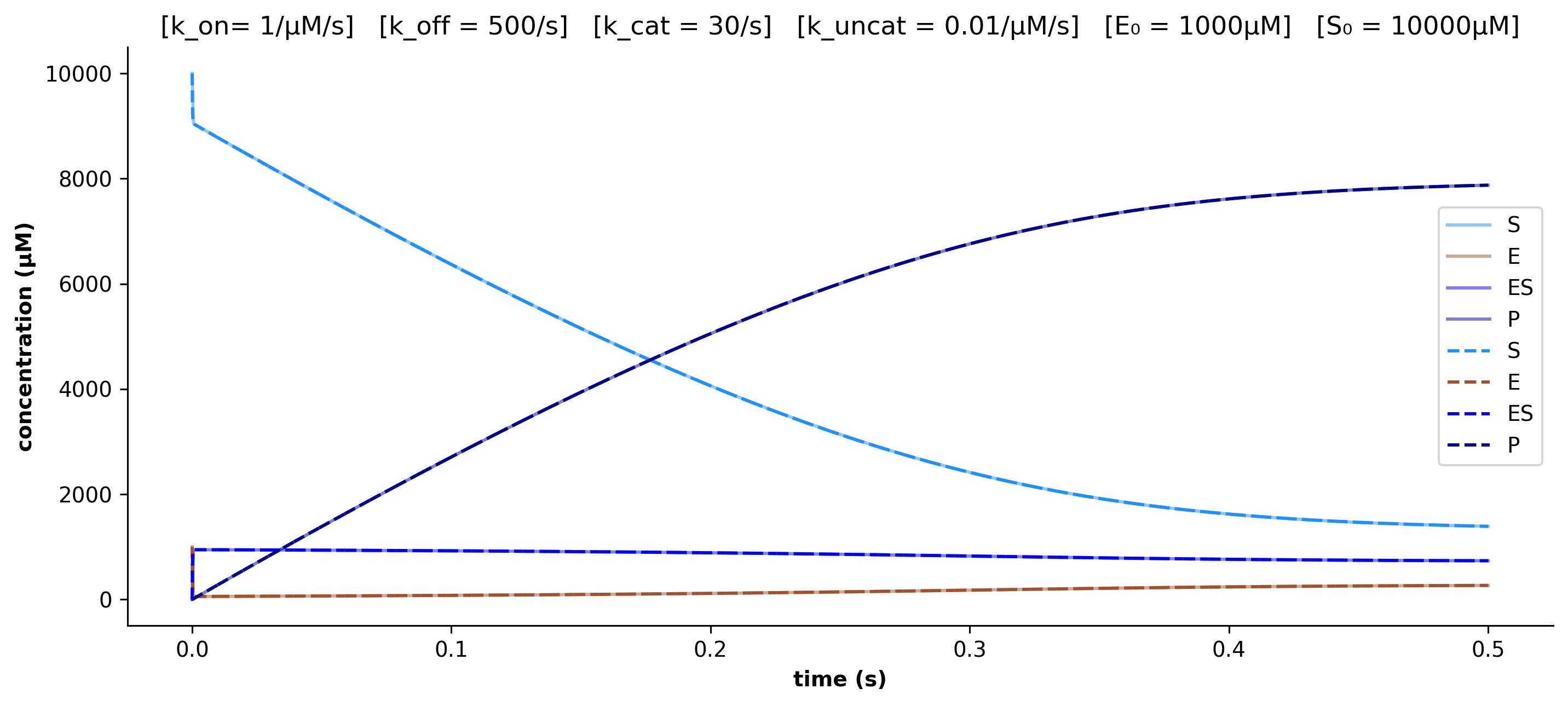# Enzyme Kinetic Parameter Inference¶

Supposing you observe a timeseries of concentrations of metabolites, can you infer the kinetic parameters for the enzyme?

• toc: true
In :
#collapse
# imports
import os, sys, pickle, time
from itertools import combinations_with_replacement, product
from collections import Counter, namedtuple
from io import StringIO
import h5py
from pathlib import Path
import numpy as np
import pandas as pd
import matplotlib.pyplot as plt
import matplotlib.ticker as mtick
import matplotlib.patches as patches
from matplotlib.colors import to_hex
import plotly.graph_objs as go
import plotly.express as px
from plotly.subplots import make_subplots
import seaborn as sns
import scipy.stats
from scipy.stats import multivariate_normal

from ipywidgets import interact, interactive, IntSlider, SelectionSlider, fixed
from IPython.display import display, clear_output

%config InlineBackend.figure_format = 'retina'
%matplotlib inline

# from IPython.display import set_matplotlib_formats
# set_matplotlib_formats('svg')

plt.rcParams['figure.figsize'] = [12, 5]
plt.rcParams['figure.dpi'] = 140
plt.rcParams['agg.path.chunksize'] = 10000
plt.rcParams['animation.html'] = 'jshtml'
plt.rcParams['hatch.linewidth'] = 0.3

exp = np.exp
sqrt = np.sqrt
Π = np.prod
π = np.pi
N = np.random.normal

def hex_to_rgb(h): return [int(h.lstrip('#')[i:i+2], 16)/256 for i in (0, 2, 4)]
matplotlib_colors = plt.rcParams['axes.prop_cycle'].by_key()['color']

import warnings
warnings.filterwarnings(action='once')

In :
#collapse
# resize figure special function
from IPython.core.display import Image, HTML
import io
import binascii

def resize_fig(width, height):

s = io.BytesIO()
plt.savefig(s, format='png', bbox_inches="tight", dpi=200)
plt.close()

return f'<img width="{width}" height="{height}" class="keep_dims" src="data:image/png;base64,{binascii.b2a_base64(s.getvalue()).decode()}&#10;">'


## Background¶

$$\newcommand{\kon}{k_{\mathrm{on}}} \newcommand{\koff}{k_{\mathrm{off}}} \newcommand{\kcat}{k_{\mathrm{cat}}} \newcommand{\kuncat}{k_{\mathrm{uncat}}} \newcommand{\kms}{k_{m,\mathrm{S}}} \newcommand{\kmp}{k_{m,\mathrm{P}}} \newcommand{\dSdt}{\frac{d[\mathrm{S}]}{dt}} \newcommand{\dEdt}{\frac{d[\mathrm{E}]}{dt}} \newcommand{\dESdt}{\frac{d[\mathrm{ES}]}{dt}} \newcommand{\dPdt}{\frac{d[\mathrm{P}]}{dt}}$$

### Enzyme Kinetics¶

Enzymes catalyze many critical chemical reactions in cells.

Describing a cell with a mathematical model (a long-standing goal of computational biologists) would entail modelling each enzyme-catalyzed chemical reaction.

However, although we may know the scheme for many enzymatic reactions (the responsible enzyme, the associated substrates, and resultant products) we are often missing many of the details needed to construct a faithful mathematical model of the reaction.

Let's begin by introducing the mathematical model used to describe enzymatic reaction schemes. Consider the following enzymatically-catalyzed (uni uni) chemical reaction scheme:

$$E+S \underset{\koff}{\overset{\kon}{\rightleftarrows}} ES \underset{\kuncat}{\overset{\kcat}{\rightleftarrows}}E+P$$

In this scheme E is an enzyme, S is its substrate, ES is the enzyme-substrate complex, which is an intermediate, and P is the product of the reaction. Each of those chemical species has a concentration in a fixed volume, which we denote with brackets (e.g. $[\mathrm{E}]$ = enzyme concentration).

If we make the simplifying assumption that the 4 molecular species are 'well-mixed' in solution, we can invoke the 'Law of Mass Action' under which the rate of each of the four included reactions is linear in the concentrations of the reactants (with an associated coefficient called the rate constant). The reactions in the above scheme are: enzyme-substrate association ($\kon$), dissociation ($\koff$), enzyme catalysis of substrate into product ($\kcat$), and enzyme-product re-association ("uncatalysis", $\kuncat$). The designation of 'substrate' and 'product' is our choice -- the model is entirely symmetric, which is reflected in the associated ODEs:

\begin{aligned} \frac{d[\mathrm{S}]}{dt} &= k_{\mathrm{off}}[\mathrm{ES}] - k_{\mathrm{on}}[\mathrm{E}][\mathrm{S}] \\ \frac{d[\mathrm{E}]}{dt} &= k_{\mathrm{off}}[\mathrm{ES}] - k_{\mathrm{on}}[\mathrm{E}][\mathrm{S}] + k_{\mathrm{cat}}[\mathrm{ES}] - k_{\mathrm{uncat}}[\mathrm{E}][\mathrm{P}] \\ \frac{d[\mathrm{ES}]}{dt} &= - k_{\mathrm{off}}[\mathrm{ES}] + k_{\mathrm{on}}[\mathrm{E}][\mathrm{S}] - k_{\mathrm{cat}}[\mathrm{ES}] + k_{\mathrm{uncat}}[\mathrm{E}][\mathrm{P}] \\ \frac{d[\mathrm{P}]}{dt} &= k_{\mathrm{cat}}[\mathrm{ES}] - k_{\mathrm{uncat}}[\mathrm{E}][\mathrm{P}] \end{aligned}

This differential equation model describing the (deterministic) chemical kinetics for an enzymatically-catalyzed reaction in well-mixed conditions contains 4 kinetic parameters, i.e. 4 degrees of freedom, which we do not know a priori. These will be the subject of inference.

Note: the intracellular environment is not best described as well-mixed, and models of 'Macromolecular Crowding' have led to more accurate rate laws for these reactions in vivo. However, we will retain the well-mixed assumption for now.

### Parameter Inference¶

There are 3 typical problems associated with ODE models:

• Supplied with a complete specification of the system, the forward problem is to integrate the differential equations from some initial conditions forwards in time and predict the trajectory of the system. This is what is typically meant by "solving" the ODE system, but exact analytical solutions are rare, and numerical methods are often brought to bear to approximate system trajectories.
• Supplied with one or more trajectories (data) but incomplete specification of the system, the inverse problem is to estimate parameters of the system (coefficients in the ODE expressions).
• Finally, given some manipulable inputs, the control problem is to drive the system towards some desired state.

This post will explore a range of approaches for the inverse problem. Our goal will be to estimate the kinetic parameters of enzymatically-catalyzed chemical reactions from timeseries of concentrations of the molecular species.

Note: enzyme kinetic parameters are typically not inferred from metabolite timeseries data using the methods we will describe, but instead from specific enzyme assays. However, at the moment, these assays are limited to studying one enzyme at a time. The inference approaches described in this post can leverage data from emerging high-throughput assays, which measure many molecular concentrations at once.

The determination of the kinetic parameters for the enzymatic reactions of life is a major project, and reported values have been tabulated in databases such as BRENDA. However, my experience with these databases has been that the reported kinetic parameters are not internally consistent.

### The Michaelis-Menten/Briggs-Haldane Approximation¶

Two assumptions commonly made at this point are:

1. to assume the initial substrate concentration is much larger than the enzyme concentration ($[\mathrm{S_0}] \gg [\mathrm{E_0}]$).
2. to suppose that the rates of enzyme-substrate association ($\kon$) and dissociation ($\koff$) are greater than the rates of catalysis and uncatalysis (i.e. $\kon$, $\koff$ $\gg$ $\kcat$, $\kuncat$).

These assumptions permit a timescale separation argument called the "Quasi-Steady-State Approximation" (QSSA) in which we set $\dESdt = 0$, which enables the derivation of the traditional Reversible Michaelis-Menten/Briggs-Haldane expression:

\begin{aligned} \frac{d[\mathrm{P}]}{dt} &= \frac{ \frac{\kcat \, [\mathrm{E_T}] [\mathrm{S}]}{K_{m,\mathrm{S}}} - \frac{\koff \, [\mathrm{E_T}] [\mathrm{P}]}{K_{m,\mathrm{P}}}} {1+\frac{[\mathrm{S}]}{K_{m,\mathrm{S}}} + \frac{[\mathrm{P}]}{K_{m,\mathrm{P}}}} \\ \\ \frac{d[\mathrm{S}]}{dt} &= -\frac{d[\mathrm{P}]}{dt} \end{aligned}

in which we have introduced the "Michaelis Constants": $K_{m,\mathrm{S}} = \frac{\koff + \kcat}{\kon}$ and $K_{m,\mathrm{P}} = \frac{\koff + \kcat}{\kuncat}$.

The QSSA reduces the system from 4 variables to 2. However, there are still 4 kinetic parameters to estimate in this reduced model.

Note: another assumption typically made at this point is to assume that catalysis is irreversible ($\kuncat = 0$), leading to a further simplified expression for the rate of product formation $\frac{d[\mathrm{P}]}{dt}$. However, this assumption is quite often inaccurate, so we will not make it.

## Exploring the Forward Model¶

### A Standard Example¶

Before we explore techniques to estimate enzyme kinetic parameters from timeseries data, we need to generate timeseries data to begin with. We can accomplish that by fixing kinetic parameters, then solving the forward problem. It will turn out that integrating the differential equations forwards is a subroutine of both approaches to the inverse problem we'll see in this post, so developing a method for the forward problem is hardly wasted effort.

In order to produce a trajectory, we need to set initial conditions. We'll integrate the reaction kinetics of a hypothetical in vitro experiment, in which a fixed quantity of enzyme and substrate are added to the reaction at the outset, then left to react.

Note: in vivo we would expect the concetration of enzyme to vary over time, and the substrate to be replenished. We will generalize this approach to a more biologically-relevant setting in a future post.

Our initial conditions are:

• $[E]_0$, the initial enzyme concentration, is set to 1mM (miliMolar, i.e. 1000μM).
• $[S]_0$, the initial substrate concentration is set to 10mM.
In :
default_initial_conditions = {
'S_0': 10e3,
'E_0': 1e3,
'ES_0': 0.0,
'P_0': 0.0
}


Next, let's fix some generic rate constants:

• $\kon \,$ of $10^6$ events per Mol per second, or 1 per μM per second, is a typical rate for enzyme-substrate binding.
• $\koff \,$ of 500/s results in a $\koff$/$\kon$ = $k_d$ of 500 μM, which is a typical $k_d$.
• $\kcat \,$ is 30/s, a fairly slow but respectable $\kcat$.
• $\kuncat \,$ of $\frac{\kon}{10}$ is often considered as the boundary for the QSSA to hold (so 0.1 per μM per second). Let's use $\kuncat = \frac{\kon}{100} =$ 0.01/μM for good measure.

Our units are μM and seconds.

In :
# Set default kinetic parameters
default_kinetic_params = {
'k_on': 1,
'k_off': 500,
'k_cat': 30,
'k_uncat': 0.01
}

def k_ms(p): return (p['k_off'] + p['k_cat']) / p['k_on']
def k_mp(p): return (p['k_off'] + p['k_cat']) / p['k_uncat']

default_kinetic_params['k_ms'] = k_ms(default_kinetic_params)
default_kinetic_params['k_mp'] = k_mp(default_kinetic_params)


To simulate the kinetics with little derivative steps, we need a step size, and a number of total steps:

In :
dt = 1e-6
steps = 5e5


There are a variety of numerical methods to integrate systems of differential equations. The most straightforward is Euler's method, which we've written down explicitly for this system below:

In :
# define integrate_euler_full(), which integrates the full kinetics with Euler's Method, and returns a trajectory
def integrate_euler_full(kinetic_params, dt=dt, steps=steps, initial_conditions=default_initial_conditions):

S, E, ES, P = initial_conditions.values()
k_on, k_off, k_cat, k_uncat, k_ms, k_mp = kinetic_params.values()
traj = [[S, E, ES, P]]

for _ in range(int(steps)):

dS = k_off * ES - k_on * E * S
dE = k_off * ES - k_on * E * S + k_cat * ES - k_uncat * E * P
dES = k_on * E * S - k_off * ES - k_cat * ES + k_uncat * E * P
dP = k_cat * ES - k_uncat * E * P

S += dS * dt
E += dE * dt
ES += dES * dt
P += dP * dt

traj.append([S, E, ES, P])

return pd.DataFrame(traj, columns=['S', 'E', 'ES', 'P'], index=np.around(np.linspace(0, dt*steps, int(steps)+1), 6))


We'll also write down Euler's method for the Michaelis-Menten/Briggs-Haldane kinetics

In :
#collapse
# define integrate_euler_MM(), which integrates the Michaelis-Menten/Briggs-Haldane kinetics
def integrate_euler_MM(kinetic_params, dt=dt, steps=steps, initial_conditions=default_initial_conditions):

S, E, ES, P = initial_conditions.values()
k_on, k_off, k_cat, k_uncat, k_ms, k_mp = kinetic_params.values()
traj = [P]

for _ in range(int(steps)):

dP = ((k_cat * E * S) / k_ms - (k_off * E * P) / k_mp) / (1 + S / k_ms + P / k_mp)
dS = -dP

P += dP * dt
S += dS * dt

traj.append(P)

return pd.Series(traj, name='P_MM', index=np.around(np.linspace(0, dt*steps, int(steps)+1), 6)).to_frame()


Now we can integrate the reaction kinetics, and plot the trajectory. We'll overlay the Michaelis-Menten/Briggs-Haldane kinetics with dotted lines on top of the full kinetics (solid).

In :
traj_euler_full = integrate_euler_full(default_kinetic_params)
traj_euler_mm = integrate_euler_MM(default_kinetic_params)

In :
#collapse
# figure styles
def fig_style(ax):
for side in ["right","top"]: ax.spines[side].set_visible(False)
ax.set_xlabel('time (s)',  weight='bold')
ax.set_ylabel('concentration (μM)',  weight='bold')

def param_string(E_0=None, S_0=None, k_on=None, k_off=None, k_cat=None, k_uncat=None, k_ms=None, k_mp=None, **kwargs):
return f'[k_on= {k_on}/μM/s]   [k_off = {k_off}/s]   [k_cat = {k_cat}/s]   [k_uncat = {k_uncat}/μM/s]   [E₀ = {int(E_0)}μM]   [S₀ = {int(S_0)}μM]'

c = {
'S': 'dodgerblue',
'E': 'sienna',
'ES': 'blue',
'P': 'darkblue',
'S_MM': 'steelblue',
'P_MM': 'slateblue',
'k_on': 'mediumseagreen',
'k_off': 'olive',
'k_cat': 'darkgreen',
'k_uncat': 'darkgoldenrod',
'k_m': 'olivedrab',
'k_ms': 'forestgreen',
'k_mp': 'darkkhaki',
}

c = {k:to_hex(v) for k,v in c.items()}

def color(columns): return [c[col] for col in columns]

In :
#collapse
# plot the integrated kinetics
ax = traj_euler_full.plot.line(title=param_string(**default_initial_conditions, **default_kinetic_params), color=color(traj_euler_full.columns))
traj_euler_mm.plot.line(ax=ax, color=color(traj_euler_mm.columns), linestyle='--')

fig_style(ax)We can plainly see the validity of the Quasi-Steady-State Approximation (QSSA) in action in the trajectory: Enzyme E and Substrate S rapidly form Enzyme-Substrate complex ES, the concentration of which remains relatively constant throughout the course of the reaction (recall the QSSA is the approximation that $\dESdt = 0$). Thus, the Michaelis-Menten/Briggs-Haldane product concentration trajectory P_MM well approximates the full kinetics trajectory for the concentration of product P, since the requisite assumptions are valid, namely, (1) $[\mathrm{S_0}] \gg [\mathrm{E_0}]$ and (2) $\kon$, $\koff$ $\gg$ $\kcat$, $\kuncat$.

In practice, Michaelis-Menten/Briggs-Haldane kinetics are often assumed by default, risking the possibility of their misapplication. Let's take this opportunity to explore how the MM/BH kinetics diverge from the full kinetics when we violate the requisite assumptions.

### Breaking the Michaelis-Menten/Briggs-Haldane Assumptions: Initial Substrate:Enzyme Ratio¶

Suppose first the number of molecules of substrate is not much greater than the number of molecules of enzyme, which is a plausible regime for certain reactions in vivo.

In :
# Set initial enzyme and substrate concentrations: 2 molecules of substrate for each molecule of enzyme.
initial_conditions = {
'S_0': 2e3,
'E_0': 1e3,
'ES_0': 0.0,
'P_0': 0.0
}

In :
#collapse
traj_euler_full_2 = integrate_euler_full(default_kinetic_params, steps=2e5, initial_conditions=initial_conditions)
traj_euler_mm_2 = integrate_euler_MM(default_kinetic_params, steps=2e5, initial_conditions=initial_conditions)

ax = traj_euler_full_2.plot.line(title=param_string(**initial_conditions, **default_kinetic_params), color=color(traj_euler_full_2.columns))
traj_euler_mm_2.plot.line(ax=ax, color=color(traj_euler_mm_2.columns), linestyle='--')

fig_style(ax)Then P_MM worsens significantly as an estimate of P.

### Breaking the Michaelis-Menten/Briggs-Haldane Assumptions: Fast Enzyme-Substrate Complex Kinetics¶

Suppose further that the rates of association and dissociation of enzyme with subtstrate are not substantially faster than those of enzyme and product.

In :
kinetic_params = {
'k_on': 0.05,
'k_off': 1,
'k_cat': 50,
'k_uncat': 0.5
}

kinetic_params['k_ms'] = k_ms(kinetic_params)
kinetic_params['k_mp'] = k_mp(kinetic_params)

In :
#collapse
traj_euler_full_3 = integrate_euler_full(kinetic_params, initial_conditions=initial_conditions)
traj_euler_mm_3 = integrate_euler_MM(kinetic_params, initial_conditions=initial_conditions)

ax = traj_euler_full_3.plot.line(title=param_string(**initial_conditions, **kinetic_params), color=color(traj_euler_full_3.columns))
traj_euler_mm_3.plot.line(ax=ax, color=color(traj_euler_mm_3.columns), linestyle='--')

fig_style(ax)Then the Michaelis-Menten/Briggs-Haldane kinetics diverge further.

In each of these latter trajectories, the criteria to make the Michaelis-Menten/Briggs-Haldane approximation are violated, leading to poor approximations to the full kinetics. We belabor this point here because in the following, we will seek to infer the parameters of the kinetics, and our inference will fit poorly if we fit to inappropriate kinetic expressions.

### Comparing Integrators¶

All of the above trajectories are generated by Euler's Method, the most intuitive ODE integration technique. Unfortunately, Euler's Method's naïvete has drawbacks:

• The order of the error is large with respect to the timestep size.
• The method is slow, due to the uniformity of the timestep sizes.

A variety of faster and more accurate (albeit more complicated) integrators have been proposed, many of which have implementations in scipy's integrate package. Due to their superior speeds and accuracies, we'll use these methods during inference. As a sanity check, we compare our basic Euler Method solver to scipy's:

In :
#collapse
# define integrate_scipy_full and scipy_MM integrate_scipy_MM (and helpers) to integrate chemical kinetics with scipy
from scipy.integrate import solve_ivp

def dy_full(t, y, k_on, k_off, k_cat, k_uncat, *args):
# Y ordered S,E,ES,P
dy = np.zeros(4)
dy = k_off * y - k_on * y * y
dy = k_off * y - k_on * y * y + k_cat * y - k_uncat * y * y
dy = k_on * y * y - k_off * y - k_cat * y + k_uncat * y * y
dy = k_cat * y - k_uncat * y * y
return dy

def dy_MM(t, y, S_0, E_0, ES_0, P_0, k_on, k_off, k_cat, k_uncat, k_ms, k_mp):
# Y ordered S,P
dy = np.zeros(2)
dy = ((k_cat * E_0 * y) / k_ms - (k_off * E_0 * y) / k_mp) / (1 + y / k_ms + y / k_mp)
dy = -dy

return dy

def integrate_scipy_full(kinetic_params, initial_conditions=default_initial_conditions, dt=dt, steps=steps, atol=1e-6):

t_span = (0, dt*steps)
t_eval = np.around(np.linspace(t_span,t_span,1001), decimals=5)
y0 = list(initial_conditions.values())

try:
sol = solve_ivp(dy_full, t_span, y0, args=(*kinetic_params.values(),), t_eval=t_eval, first_step=dt, method='LSODA', atol=atol)
return pd.DataFrame(sol.y.T, index=sol.t, columns=['S', 'E', 'ES', 'P'])
except:
return pd.DataFrame(columns=['S', 'E', 'ES', 'P'])

def integrate_scipy_MM(kinetic_params, initial_conditions=default_initial_conditions, dt=dt, steps=steps):

t_span = (0, dt*steps)
t_eval = np.around(np.linspace(t_span,t_span,1001), decimals=5)
y0 = [initial_conditions['S_0'], initial_conditions['P_0']]

try:
sol = solve_ivp(dy_MM, t_span, y0, args=(*initial_conditions.values(), *kinetic_params.values()), t_eval=t_eval, first_step=dt)
return pd.DataFrame(sol.y.T, index=sol.t, columns=['S_MM', 'P_MM'])
except:
return pd.DataFrame(columns=['S_MM', 'P_MM'])

In :
#collapse
# solve the system with both integrators, for default parameters
traj_scipy_full = integrate_scipy_full(default_kinetic_params)

ax = traj_scipy_full.plot.line(title=param_string(**default_initial_conditions, **default_kinetic_params), color=color(traj_scipy_full.columns), alpha=0.5)
traj_euler_full.plot.line(ax=ax, color=color(traj_euler_full.columns), linestyle='--')

fig_style(ax)In :
#collapse
# solve the system with both integrators, for unusual parameters
kinetic_params = {
'k_on': 0.02,
'k_off': 5,
'k_cat': 10,
'k_uncat': 0.00001,
}

kinetic_params['k_ms'] = k_ms(kinetic_params)
kinetic_params['k_mp'] = k_mp(kinetic_params)

start = time.process_time()
traj_euler_full_4 = integrate_euler_full(kinetic_params, initial_conditions=initial_conditions)
euler_time = time.process_time() - start

start = time.process_time()
traj_scipy_full_4 = integrate_scipy_full(kinetic_params, initial_conditions=initial_conditions)
scipy_time = time.process_time() - start

ax = traj_scipy_full_4.plot.line(title=param_string(**initial_conditions, **kinetic_params), color=color(traj_scipy_full_4.columns), alpha=0.5)
traj_euler_full_4.plot.line(ax=ax, color=color(traj_euler_full_4.columns), linestyle='--')

fig_style(ax)